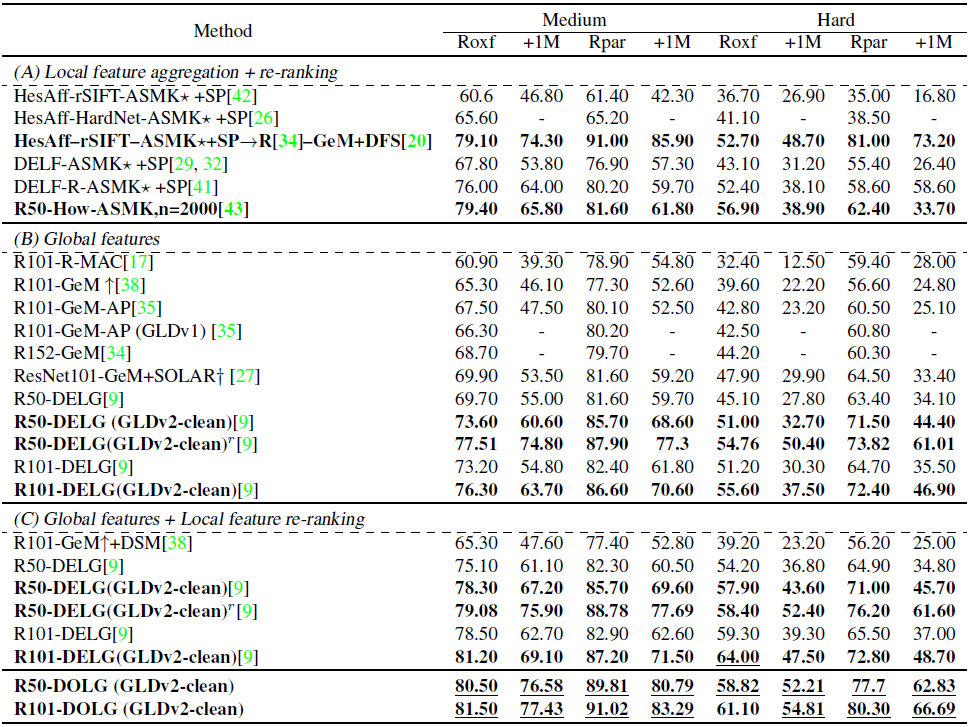DOLG Single Stage Image Retrieval with Deep Orthogonal Fusion of Local and Global Features

# 0x01 背景知识

## 0x00 GeM池化

$K\times h\times w$
$K$

$\mathcal{X}$

$\text{f}^{(g)}=[\text{f}^{(g)}_1 \cdots\text{f}^{(g)}_k\cdots\text{f}^{(g)}_K] \\ \text{where }\text{f}^{(g)}_k=(\frac{1}{|\mathcal{X}_k|}\sum_{x\in \mathcal{X}_k}x^{p_k})^{\frac{1}{p_k}},~ |\mathcal{X}_k|=h\times w$
$p_k=1$

$p_k\rightarrow \infty$

$p_k$

## 0x01 空洞卷积

$3\times3$
，dilation rate为2的卷积核：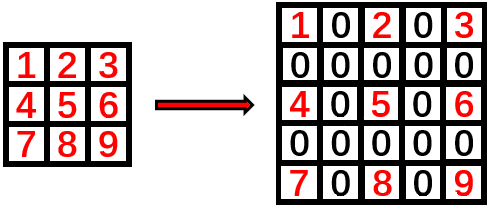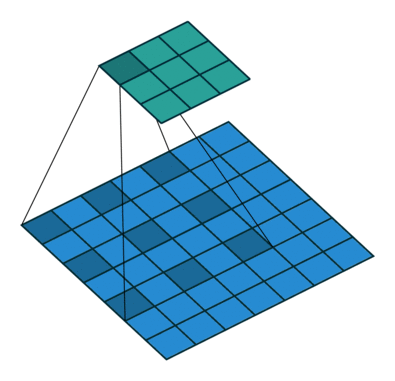## 0x02 Batch Normalization

$y=\frac{x-mean(x)}{\sqrt{Var(x)}+eps}\gamma+\beta$

$\gamma$
$\beta$

# 0x02 方法论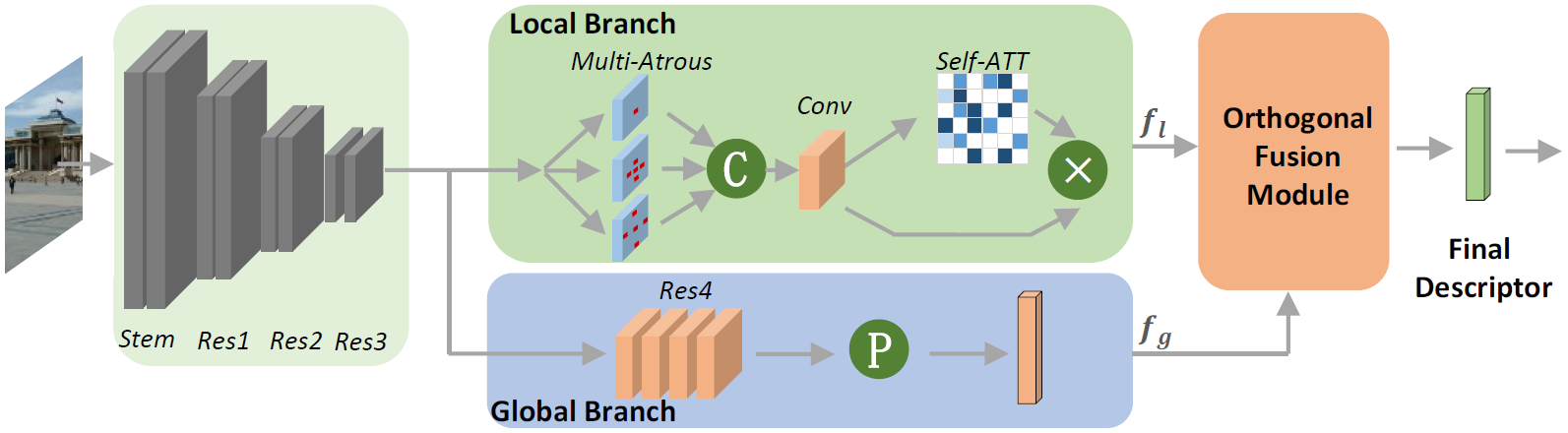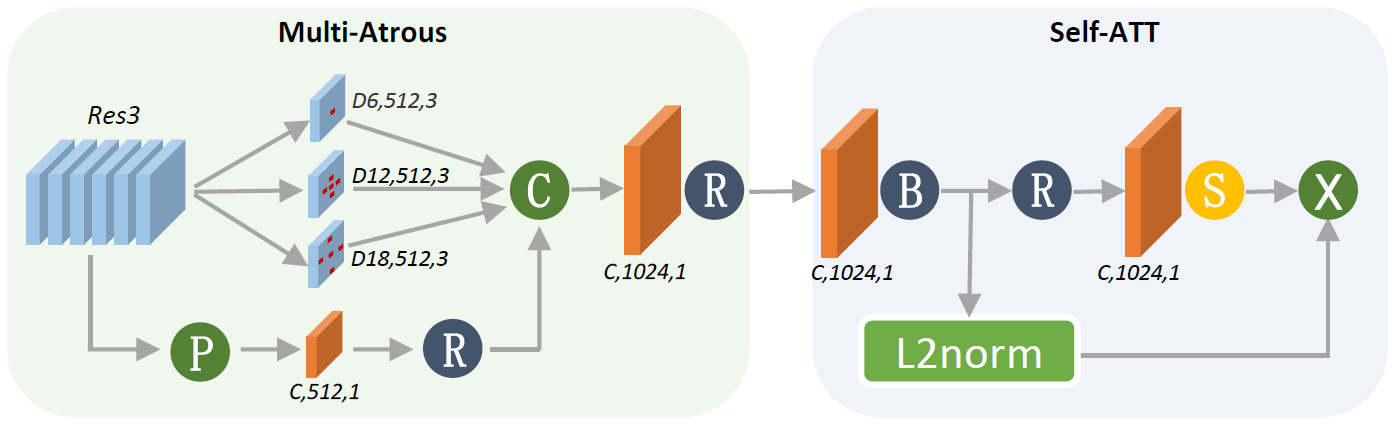$1\times 1$

ASPP的输出特征将会注入到自注意力模型（self-attention module）中来确定特征图中的关键点并输出。该模型会对每一个特征点返回一个注意力分数，将这个注意力分数与L2归一化后的特征相乘计算出来的最终结果则为该图像的局部特征。

$f_l$

$f_g$

$f_l^{(i,j)}$

$f_g$

$f_{l,proj}^{(i,j)}$
，此后其正交融合特征
$f_{l,orth}^{(i,j)}$

$f_l^{(i,j)}-f_{l,proj}^{(i,j)}$
，也就是下图中的橙色向量。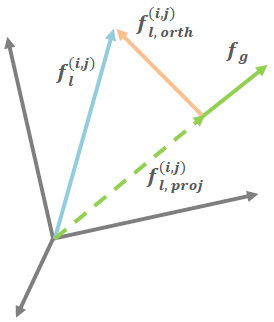$f_{l,proj}^{(i,j)}$

$f_{l,proj}^{(i,j)}=\frac{f_l^{(i,j)}\cdot f_g}{|f_g|^2}f_g$

$f_l^{(i,j)}\cdot f_g=\sum_{c=1}^Cf_{l,c}^{(i,j)}f_{g,c}\\ |f_g|^2=\sum_{c=1}^C(f_{g,c})^2$

$C\times H\times W$

$f_l$
，以及一个
$C\times1$

$f_g$
，最终得出来的融合特征
$f_{orth}$

$C\times H\times W$
$C\times 1$

$f_g$

$512\times 1$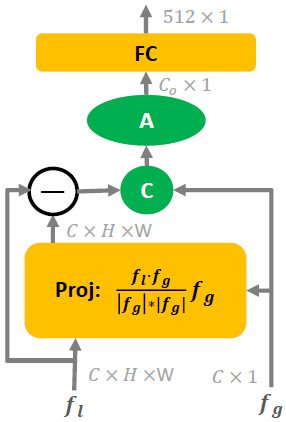$N$

$\hat{\mathcal{W}}\in R^{512\times N}$
，我们使用ArcFace损失函数来训练整个神经网络：
$L=-\log\left(\frac{\exp\left(\gamma\times AF\left(\hat\omega_t^T\hat{f_g},1\right)\right)}{\sum_n\exp\left(\gamma\times AF\left(\hat\omega_n^T\hat{f_g},y_n\right)\right)}\right)$

$\hat{f_g}$

$\hat{\mathcal{w}_i}$

$\hat{\mathcal{W}}$

$\hat{f_g}$
$\hat{\mathcal{W}}$

$\hat{\mathcal{w}_i}\hat{f_g}$

# 0x03 实践论